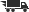Close

# Additive Number Theory the Classical Bases

## by Melvyn B. Nathanson

• ISBN: 9780387946566
• ISBN10: 038794656X

# Additive Number Theory the Classical Bases

## by Melvyn B. Nathanson

• Binding: Hardcover
• Publisher: Springer Verlag
• Publish date: 06/01/1996
• ISBN: 9780387946566
• ISBN10: 038794656X
used \$73.12
You save: 9%
new \$88.54
FREE economy shipping!
Description: The classical bases in additive number theory are the polygonal numbers, the squares, cubes, and higher powers, and the primes. This book contains many of the great theorems in this subject: Cauchy's polygonal number theorem, Linnik's theorem on sums of cubes, Hilbert's proof of Waring's problem, the Hardy-Littlewood asymptotic formula for the number of representations of an integer as the sum of positive kth powers, Shnirel'man's theorem that every integer greater than one is the sum of a bounded number of primes, Vinogradov's theorem on sums of three primes, and Chen's theorem that every sufficiently large even integer is the sum of a prime and a number that is either prime or the product of two primes. The book is also an introduction to the circle method and sieve methods, which are the principal tools used to study the classical bases. The only prerequisites for the book are undergraduate courses in number theory and analysis. Additive number theory is one of the oldest and richest areas of mathematics. This book is the first comprehensive treatment of the subject in 40 years.
Expand description
 Seller: Moe's Books ★★★★★ ★★★★★ 30 orders (93% success) Location: Berkeley, CA Condition: Very GoodPrice: \$73.12 Comments: